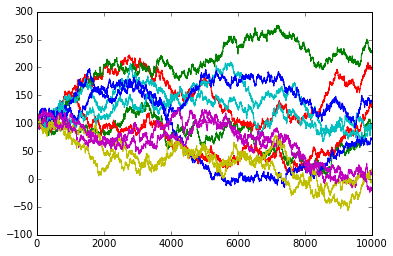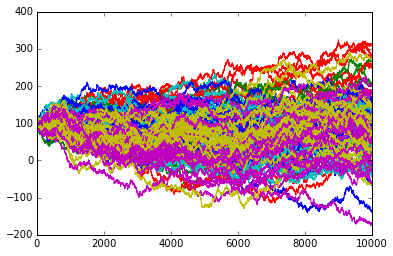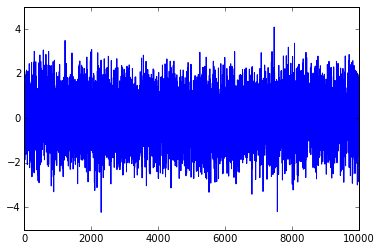# Tech Blog

## Our Data Scientists Comment on The Grapples

### A GNPR tutorial: How to cluster random walks

by Gautier MARTI, Hellebore Capital Management

Since the seminal work of Bachelier (1900), stochastic processes are extensively used to model the behaviours of financial assets. Moreover, the efficient-market hypothesis (1965) due to Eugene Fama (2013 Nobel Prize winner) points out that at least some financial markets are suitable for applying this modelling. Concerning the state-of-the-art in clustering financial assets, there is a strong focus on correlation clustering, but much less attention is paid to the underlying returns distribution. However, we may want to take this information into account when performing analysis on financial assets: what about two assets having Kendall's tau of 1 (high correlation), but only one of them undergoing fat tails? what about uncorrelated assets having nonetheless a similar returns distribution qualifying them as illiquid products? To answer these legitimate questions, we design a clustering workflow able to recover correlation clusters, distribution clusters or a mix of both. Below is a visual summary of our approach versus a more classical one recovering only correlation clusters.#### Generating correlated random walks whose increments are drawn from two distributions, namely Gaussian and Laplacian.

The function create_random_walks below creates the random walks S$S$ which are splitted into K$K$ dependence clusters, and each of these K$K$ dependence clusters are divided into two distribution clusters yielding to 2K$2K$ clusters in S$S$.

In :
import numpy as np
import scipy
from scipy import stats
from scipy.stats import mstats

def create_random_walks(rho_market,rho_cluster,K,N,T):
random_walks = np.zeros((N,T+1))

market_factor = np.random.normal(0,1,T)

cluster_factors = np.zeros((K,T))
for k in range(0,K):
cluster_factors[k,] = np.random.normal(0,1,T)

idiosync_factors = np.zeros((N,T))
for n in range(0,N):
if n%2:
idiosync_factors[n,] = np.random.normal(0,1,T)
else:
idiosync_factors[n,] = np.random.laplace(0,1/np.sqrt(2),T)

increments = np.zeros((N,T))
cluster_class = 0
size_class = np.floor(N/K)

for n in range(0,N):

market_compo = np.sqrt(rho_market)*market_factor
indus_compo = np.sqrt(rho_cluster)*cluster_factors[cluster_class,]
idiosync_compo = np.sqrt(1-rho_market-rho_cluster)*idiosync_factors[n,]
increments[n,] = market_compo + indus_compo + idiosync_compo

if n%2:
random_walks[n,T] = 2*cluster_class
else:
random_walks[n,T] = 2*cluster_class+1

if(((n+1)%size_class == 0) and (cluster_class < K-1)):
cluster_class += 1

for n in range(0,N):
random_walks[n,0] = 100
for t in range(1,T):
random_walks[n,t] = random_walks[n,t-1] + increments[n,t]

return random_walks


We illustrate random walks generated with the following parameters:

In :
rho_market = 0.1
rho_cluster = 0.1
K = 3
N = 12
T = 10000

random_walks = create_random_walks(rho_market,rho_cluster,K,N,T)


yielding to:

In :
import matplotlib.pyplot as plt
%matplotlib inline

color_map = ['r','g','b','c','m','y']
for i in range(0,N):
plt.plot(random_walks[i,:T],color=color_map[int(random_walks[i,T]%len(color_map))])
plt.show()where random walks from the same cluster share the same color. Notice that it is visually painful to identify the clustering, even for such a small number of clusters and time series. With more data, it's practically impossible!

In :
rho_market = 0.1
rho_cluster = 0.1
K = 3
N = 120
T = 10000

lotsof_random_walks = create_random_walks(rho_market,rho_cluster,K,N,T)

import matplotlib.pyplot as plt
%matplotlib inline

color_map = ['r','g','b','c','m','y']
for i in range(0,N):
plt.plot(lotsof_random_walks[i,:T],color=color_map[int(lotsof_random_walks[i,T]%len(color_map))])
plt.show()#### Differencing time series: S↦dS$S \mapsto dS$

In :
def differentiate(r_walk):
N = r_walk.shape
T = r_walk.shape - 1
dS = np.diff(r_walk[0:,:T])

return dS


Below an example of an increment time series obtained:

In :
dS = differentiate(random_walks)
plt.plot(dS[1,])
plt.show()#### Applying GNPR transform: dS↦(empirical copula coordinate,margin)$dS \mapsto (\text{empirical copula coordinate}, \text{margin})$

Now, we build an appropriate representation of our data. First, we deal with the dependence part using rank statistics:

In :
def get_dependence_repr(data):
N = data.shape
T = data.shape

return scipy.stats.mstats.rankdata(data,1)


Then, we deal with the distribution part:

In :
def get_distribution_repr(data):
N = data.shape
T = data.shape
nbBins = 200
distribution_repr = np.zeros((N,nbBins))
for i in range(0,N):
hist, bin_edges = np.histogram(data[i,], bins=nbBins, range=(-10,10), density=True)
distribution_repr[i,] = hist*np.diff(bin_edges)

return distribution_repr


We then construct the GNPR representation:

In :
def get_gnpr(data,theta):
dependence_repr = get_dependence_repr(data)
distribution_repr = get_distribution_repr(data)

N = data.shape
T = data.shape
L = dependence_repr.shape+distribution_repr.shape

dependence_dist = np.zeros((N,N))
distrib_dist = np.zeros((N,N))
for i in range(0,N):
for j in range(0,N):
dependence_dist[i,j] = np.sum(np.power((dependence_repr[i,] - dependence_repr[j,]),2)) / ((1/(3*T))*(T+1)*(T-1))
distrib_dist[i,j] = np.sum(np.power((np.sqrt(distribution_repr[i,]) - np.sqrt(distribution_repr[j,])),2)) / 2

dep_dist_max = dependence_dist.max()
distrib_dist_max = distrib_dist.max()

gnpr = np.zeros((N,L))
for i in range(0,N):
gnpr[i,0:T] = dependence_repr[i,]*np.sqrt(theta)*np.sqrt(3*T)/np.sqrt((T+1)*(T-1)*dep_dist_max)
gnpr[i,T:] = np.sqrt(distribution_repr[i,])*np.sqrt(1-theta)/np.sqrt(2*distrib_dist_max)

return gnpr


#### Applying the whole GNPR workflow:

In :
#input parameters of the random walks model
rho_market = 0.1
rho_cluster = 0.1
K = 3
N = 120
T = 10000
nbDistrib = 2
nbCluster = nbDistrib*K

#transforming raw data to GNPR
random_walks = create_random_walks(rho_market,rho_cluster,K,N,T)
dS = differentiate(random_walks)
gnpr = get_gnpr(dS,theta=0.5)

#apply a clustering algorithm
from sklearn.cluster import KMeans
from sklearn import metrics

np.random.seed(42)
kmeans = KMeans(init='k-means++', n_clusters=nbCluster, n_init=50)
kmeans.fit(gnpr)

Adjusted Rand Index: 1.0



#### Benchmarking the workflow:

GNPR can accurately recover the 2,3,6-partition whereas straightforward approach recovers only the 3-partition corresponding to correlation clusters.

In :
def get_dependence_cl(finest_class):
return np.floor(finest_class/2)
def get_distribution_cl(finest_class):
return finest_class % 2

parameters = ((nbDistrib,0,get_distribution_cl(random_walks[0:,T])), \
(K,1,get_dependence_cl(random_walks[0:,T])),\
(nbCluster,0.5,random_walks[0:,T]))
for nb_cluster, theta, benchmark in parameters:
gnpr = get_gnpr(dS,theta)
kmeans = KMeans(init='k-means++', n_clusters=nb_cluster, n_init=50)
kmeans.fit(gnpr)
print("K-Means++ on GNPR:","theta =",theta,"nb_cluster =",nb_cluster)

kmeans = KMeans(init='k-means++', n_clusters=nb_cluster, n_init=50)
kmeans.fit(dS)
print("K-Means++ on dS:","nb_cluster =",nb_cluster)

K-Means++ on GNPR: theta = 0 nb_cluster = 2
1.0
K-Means++ on dS: nb_cluster = 2
-0.00752587017873939

K-Means++ on GNPR: theta = 1 nb_cluster = 3
1.0
K-Means++ on dS: nb_cluster = 3
1.0

K-Means++ on GNPR: theta = 0.5 nb_cluster = 6
1.0
K-Means++ on dS: nb_cluster = 6
0.4731699870238913



Arthur D. and Vassilvitskii, S., 2007. k-means++: the advantages of careful seeding.
Bachelier L., 1900. Théorie de la spéculation.
Fama E.F., 1965. The Behavior of Stock-Market Prices.
Tumminello M., Lillo F., Mantegna R.N., 2010. Correlation, hierarchies, and networks in financial markets.
Ryabko D., 2010. Clustering processes.